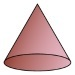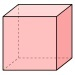##### Notes

Daily Math Practice provides a week's worth of problems ideal for morning work or review. Week 32 reviews standards from previous weeks and supports Common Core Mathematics Standards K.MD.A.1, K.MD.A.2, K.MD.B.3, K.OA.A.4, & K.OA.A.5.

##### Print Instructions

NOTE: Only your test content will print.
To preview this test, click on the File menu and select Print Preview.

See our guide on How To Change Browser Print Settings to customize headers and footers before printing.

# Kindergarten - Daily Math Practice - Week 32 (Kindergarten)

Print Test (Only the test content will print)
 DAILY MATH PRACTICE - WEEK 32 DAY 1 DAY 2 How many more squares are needed to make 10?+ 3 = 10 Add.2 + 3 =Clap the numbers. Write the answer.4 + 4 =There are 8 soccer balls in a bag. 3 balls are taken out. How many soccer balls are left in the bag?Answer:soccer balls Circle the word that describes the weight of the leaves.LIGHT or HEAVY Circle the one that holds more.Are there equal numbers of white and black squares?YES or NO Circle the solid shapes.DAILY MATH PRACTICE - WEEK 32 DAY 3 DAY 4 How many more squares are needed to make 10?+ 9 = 10 Subtract.5 - 3 =Cross of the blocks to show the equation. Write the answer.6 - 2 =Meg has 1 dog. She has 3 cats. How many cats and dogs does Meg have?Answer:cats and dogs Circle squares. Color hexagons.How many squares?How many hexagons? Circle the one that is longer.Write the missing number.10 += 18 Write the missing number.2 + 6 =+ 4You need to be a HelpTeaching.com member to access free printables.